# Joint Entity and Relation Extraction with Set Prediction Networks

## SPN

$$P(Y \mid X ; \theta)=p_{L}(n \mid X) \prod_{i=1}^{n} p\left(Y_{i} \mid X, Y_{j \neq i} ; \theta\right)$$

### Sentence Encoder

Sentence Encoder直接用BERT, 提取到的特征为$\mathbf{H}_e \in \mathbb{R} ^{l \times d}$, $l$ 为包括[CLS][SEP]的序列长度.

### Non - Autoregressive Decoder for Triple Set Generation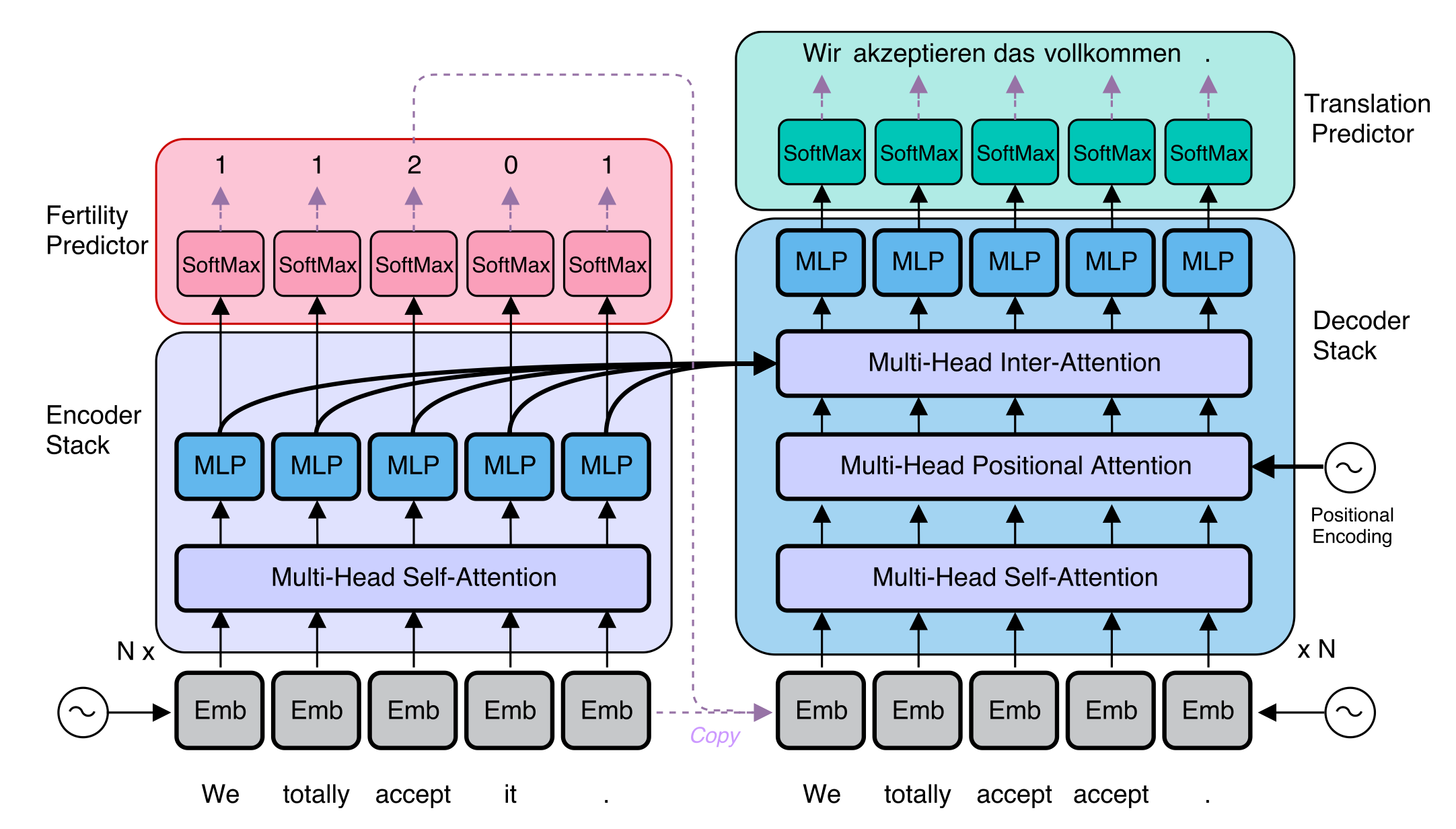#### Input

$$P(Y \mid X ; \theta)=\prod_{i=1}^{n} p\left(Y_{i} \mid X, Y_{j<i} ; \theta\right)$$

$$P(Y \mid X ; \theta)=p_{L}(n \mid X) \prod_{i=1}^{n} p\left(Y_{i} \mid X, Y_{j \neq i} ; \theta\right)$$

#### Architecture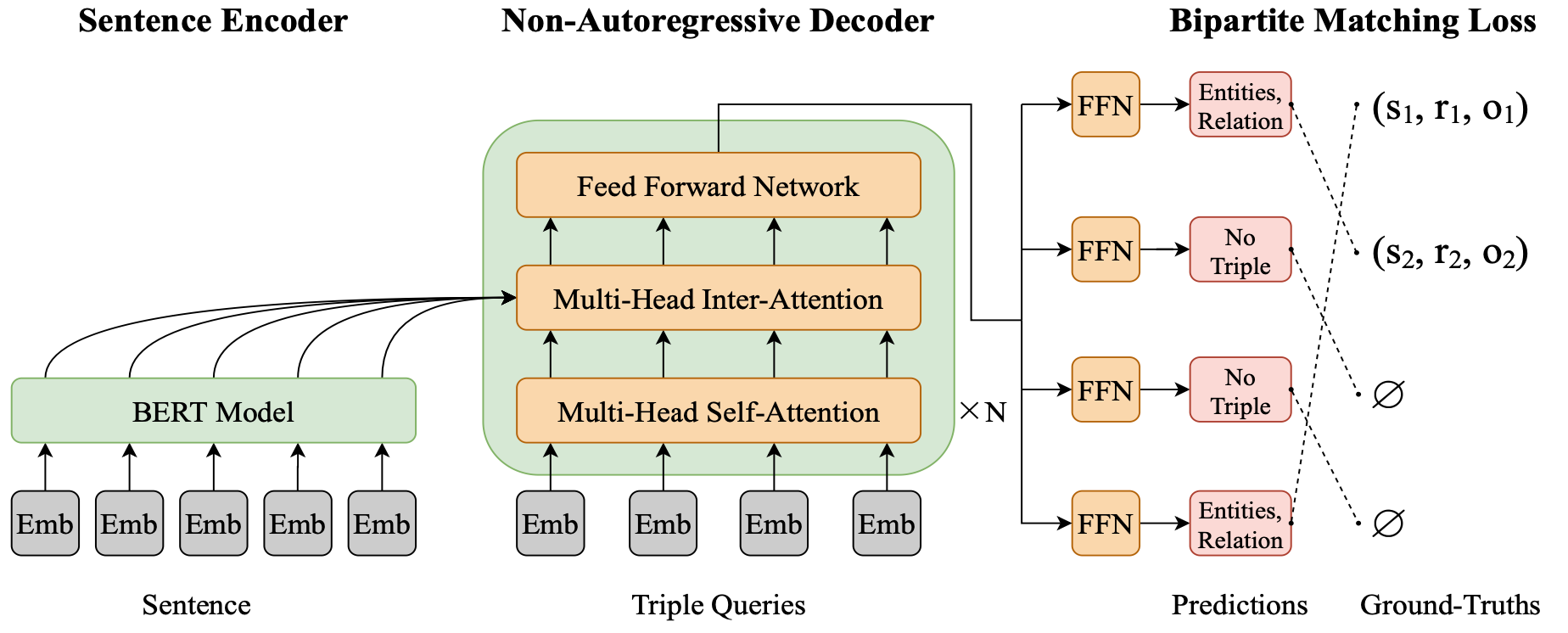$$\mathbf{p}^{r}=\operatorname{softmax}\left(\mathbf{W}_{\mathbf{r}} \mathbf{h}_{\mathrm{d}}\right)$$

\begin{aligned} \mathbf{p}^{s-s t a r t} &=\operatorname{softmax}\left(\mathbf{v}_{\mathbf{1}}^{\mathbf{T}} \tanh \left(\mathbf{W}_{\mathbf{1}} \mathbf{h}_{\mathrm{d}}+\mathbf{W}_{\mathbf{2}} \mathbf{H}_{\mathbf{e}}\right)\right) \\ \mathbf{p}^{s-e n d} &=\operatorname{softmax}\left(\mathbf{v}_{\mathbf{2}}^{\mathbf{T}} \tanh \left(\mathbf{W}_{\mathbf{3}} \mathbf{h}_{\mathrm{d}}+\mathbf{W}_{\mathbf{4}} \mathbf{H}_{\mathbf{e}}\right)\right) \\ \mathbf{p}^{o-s t a r t} &=\operatorname{softmax}\left(\mathbf{v}_{\mathbf{3}}^{\mathbf{T}} \tanh \left(\mathbf{W}_{\mathbf{5}} \mathbf{h}_{\mathrm{d}}+\mathbf{W}_{\mathbf{6}} \mathbf{H}_{\mathbf{e}}\right)\right) \\ \mathbf{p}^{o-e n d} &=\operatorname{softmax}\left(\mathbf{v}_{\mathbf{4}}^{\mathbf{T}} \tanh \left(\mathbf{W}_{\mathbf{7}} \mathbf{h}_{\mathrm{d}}+\mathbf{W}_{\mathbf{8}} \mathbf{H}_{\mathbf{e}}\right)\right) \end{aligned}

### Bipartite Matching Loss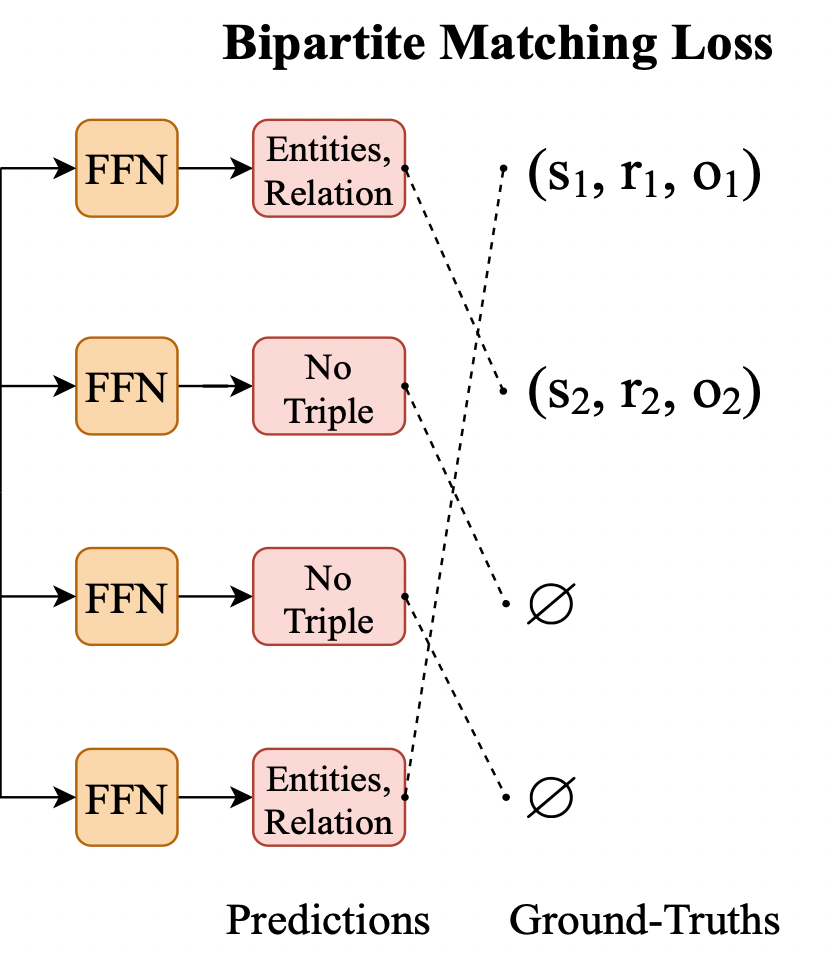$$\pi^{\star}=\underset{\pi \in \Pi(m)}{\arg \min } \sum_{i=1}^{m} \mathcal{C}_{m a t c h}\left(\mathbf{Y}_{i}, \hat{\mathbf{Y}}_{\pi(i)}\right)$$

\begin{aligned} \mathcal{C}_{\text {match}}\left(\mathbf{Y}_{i}, \hat{\mathbf{Y}}_{\pi(i)}\right) &=-\mathbb{1}_{\left\{r_{i} \neq \varnothing\right\}}\left[\mathbf{p}_{\pi(i)}^{r}\left(r_{i}\right)\right.\\ &+\mathbf{p}_{\pi(i)}^{s-start}\left(s_{i}^{start}\right) \\ &+\mathbf{p}_{\pi(i)}^{s-e n d}\left(s_{i}^{end }\right) \\ &+\mathbf{p}_{\pi(i)}^{o- start }\left(o_{i}^{start }\right) \\ &+\mathbf{p}_{\pi(i)}^{o-e n d}\left(o_{i}^{end }\right)\left.\right] \end{aligned}

\begin{aligned} \mathcal{L}(\mathbf{Y}, \hat{\mathbf{Y}}) &=\sum_{i=1}^{m}\left\{-\log \mathbf{p}_{\pi^{\star}(i)}^{r}\left(r_{i}\right)\right.\\ &+\mathbb{1}_{\left\{r_{i} \neq \varnothing\right\}}\left[-\log \mathbf{p}_{\pi^{\star}(i)}^{s-s t a r t}\left(s_{i}^{start }\right)\right.\\ &-\log \mathbf{p}_{\pi^{\star}(i)}^{s-e n d}\left(s_{i}^{end}\right) \\ &-\log \mathbf{p}_{\pi^{\star}(i)}^{o-s t a r t}\left(o_{i}^{start }\right) \\ &\left.\left.-\log \mathbf{p}_{\pi^{\star}(i)}^{o-e n d}\left(o_{i}^{end}\right)\right]\right\} \end{aligned}

## Experiments

### Datasets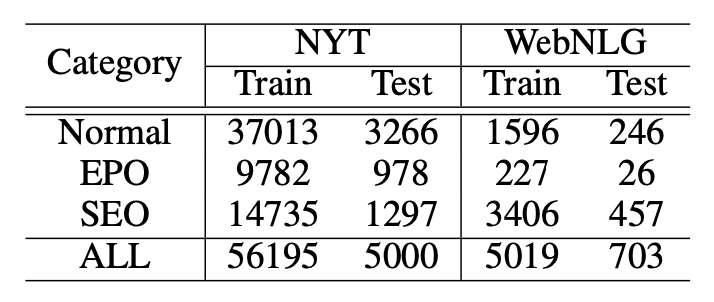### Main Results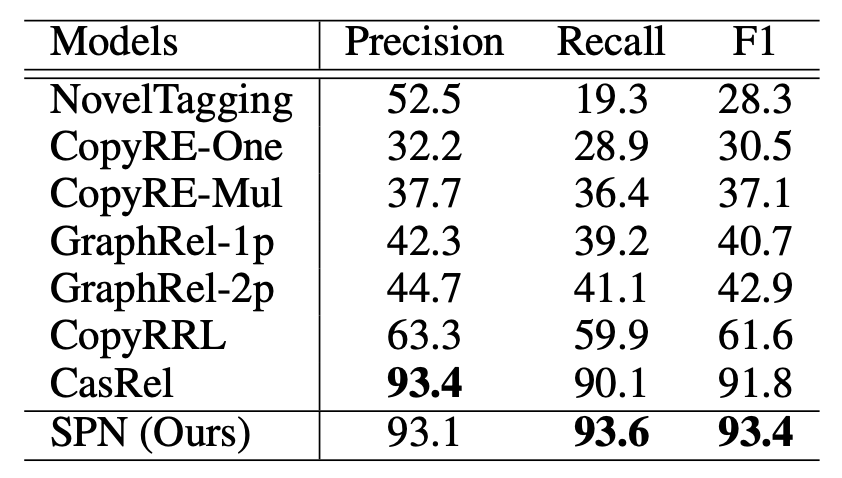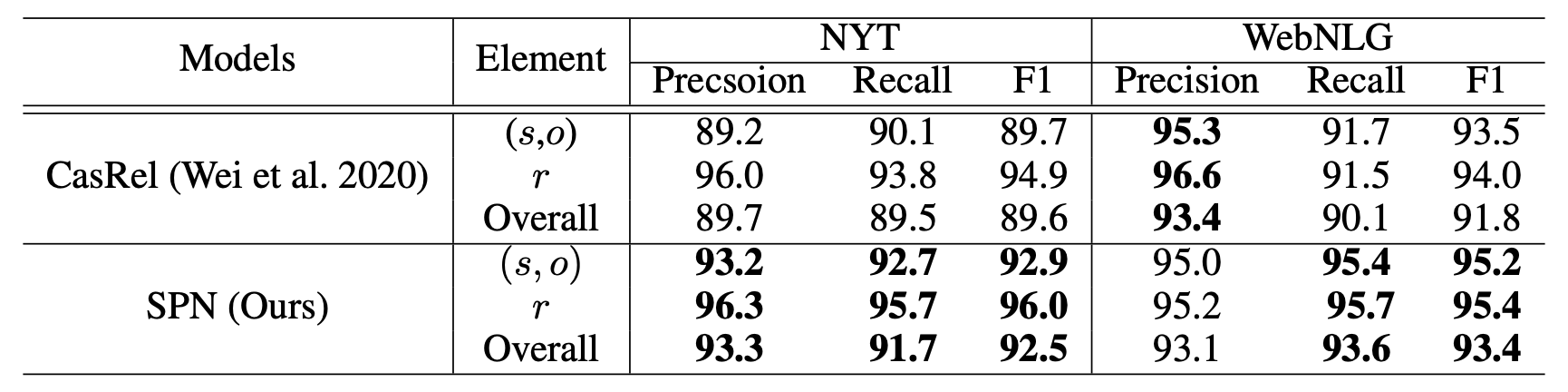CasRel除了在WebNLG的精度上稍稍比SPN好一点, 其余地方都不如SPN.

### Ablation Study

SPN在NYT24上的消融实验结果如下: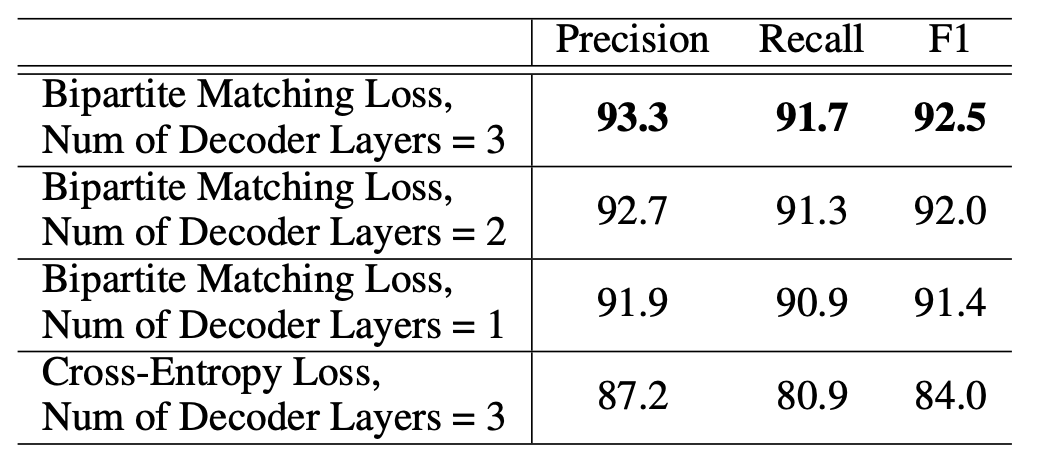### Sentences with Different Number of Triples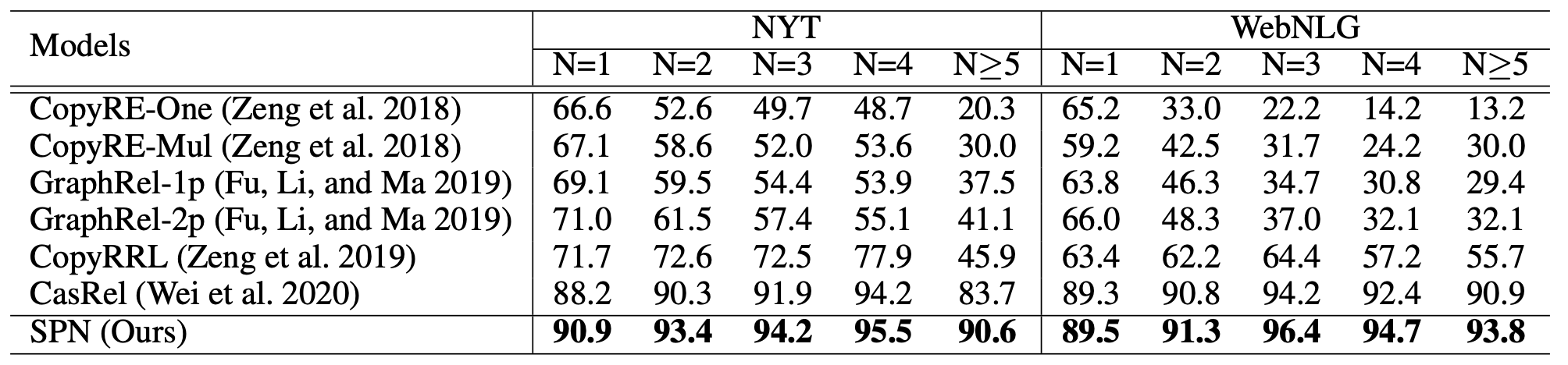SPN的各类型抽取效果都比CasRel好, 在NYT上的多三元组抽取比CasRel好挺多.

### Different Overlapping Patterns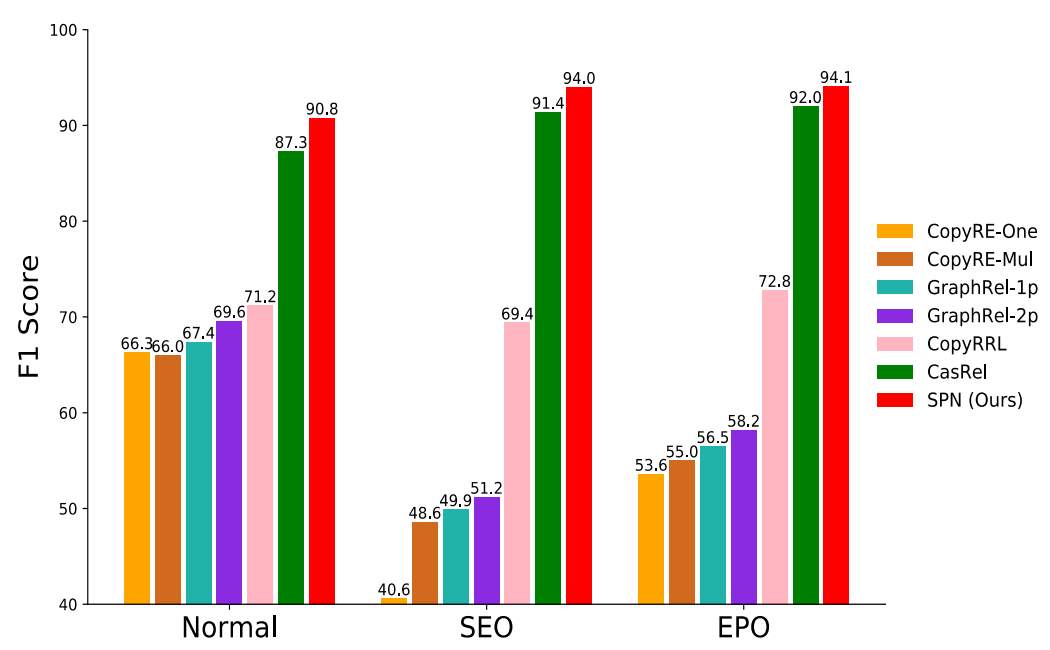SPN本身并没有设计特别针对SEO和EPO的优化方法, 似乎对普通三元组的抽取能力提升更大些.

## Summary

SPN算是生成Tagging混合的RTE模型, 不同于前人所使用的生成式方法, 将三元组抽取完全建模为自回归生成式任务, 而是将RTE建模为集合预测问题, 避免了三元组生成时存在的先后顺序问题, 同时因为使用了NAD, 获得了双向解码的能力, 使得每个三元组生成时都可以考虑到其他三元组的信息.

上一篇RFBFN: A Relation - First Blank Filling Network for Joint Relational Triple Extraction

2022-07-09GRTE: A Novel Global Feature-Oriented Relational Triple Extraction Model based on Table Filling

2022-06-02
目录